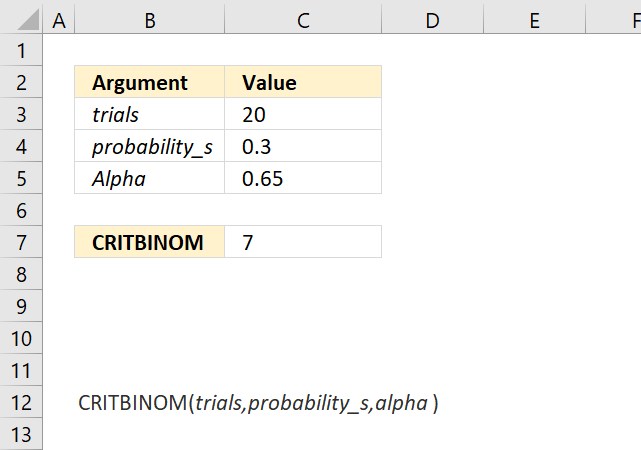Author: Oscar Cronquist Article last updated on October 08, 2018The CRITBINOM function calculates the minimum value for which the binomial distribution is equal to or greater than a given threshold value.

The CRITBINOM function is outdated and has been replaced with the BINOM.INV function.

Formula in cell C8:

=CRITBINOM(C3,C4,C5)

### Excel Function Syntax

CRITBINOM(trials,probability_s,alpha)

### Arguments

 trials Required. How many Bernoulli trials. probability_s Required. The probability of success in each test. Alpha Required. The threshold value.

### Comments

The CRITBINOM function returns

• #VALUE! error value if any argument is non-numeric.
• #NUM! error value if:
• trials < 0 (zero)
• probability_s < 0 (zero)
• alpha < 0 (zero)
• alpha > 1